﻿ 20-sim webhelp > Library > Signal > Transfer Functions > TransferFunction

# TransferFunction

## Library

Signal\Transfer Functions

FO

FOL

FOLA

SOI

SOO

SOU

SOUO

SOOL

## Use

Domains: Discrete, Continuous. Size: 1-D. Allowed in: Block Diagrams.

## Description - FO

This model describes a first order process determined by k and tau. The transfer function of this process is: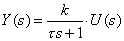Inputs

Description

input

output

## Initial Values

state_initial

The initial value of the output.

## Parameters

k

tau

Proportional gain [].

Process time constant [s].

## Description - FOL

This model describes a first order process with lead time determined by the parameters k, tau and tauL. The transfer function of this process is: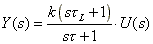Inputs

Description

input

output

state_initial

Internal state.

## Parameters

k

tau

tauL

Proportional gain [].

Process time constant [s].

## Description - FOLA

This model describes a first order process with lag determined by the parameters k, tau and tauL. The transfer function of this process is: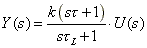Inputs

Description

input

output

state_initial

Internal state.

## Parameters

k

tau

tauL

Proportional gain [].

Process time constant [s].

Lag time constant [s].

## Description - SOI

This model describes a second order process with integrator determined by the parameters k and tau. The transfer function of this process is: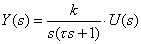Inputs

Description

input

output

## Initial Values

state1_initial

state2_initial

Internal state.

The initial value of the output.

## Parameters

k

tau

Proportional gain [].

Process time constant [s].

## Description - SOO

This model describes an overdamped second order process determined by the parameters k, tau1 and tau2. The transfer function of this process is: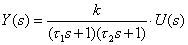Inputs

Description

input

output

## Initial Values

state1_initial

state2_initial

Internal state.

The initial value of the output.

## Parameters

k

tau1

tau2

Proportional gain [].

First process time constant [s].

Second process time constant [s].

## Description - SOU

This model describes an underdamped second order process, determined by the parameters k, tau and zeta. The transfer function of this process is: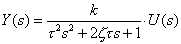Inputs

Description

input

output

## Initial Values

state1_initial

state2_initial

Internal state.

The initial value of the output after the dead time has passed.

## Parameters

k

tau

zeta

Proportional gain [].

Natural period of oscillation [s].

Damping factor [].

## Description - SOUO

This model describes an underdamped second order process, determined by the parameters k, omega and zeta. The transfer function of this process is: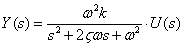Inputs

Description

input

output

## Initial Values

state1_initial

state2_initial

Internal state.

The initial value of the output after the dead time has passed.

## Parameters

k

omega

zeta

Proportional gain [].

Damping factor [].

## Description - SOOL

This model describes an overdamped second order process with lead time determined by the parameters k, tau1, tau2 and tauL. The transfer function of this process is: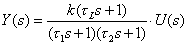Inputs

Description

input

output

## Initial Values

state1_initial

state2_initial

Internal state.

The initial value of the output.

## Parameters

k

tau1

tau2

tauL

Proportional gain [].

First process time constant [s].

Second process time constant [s].